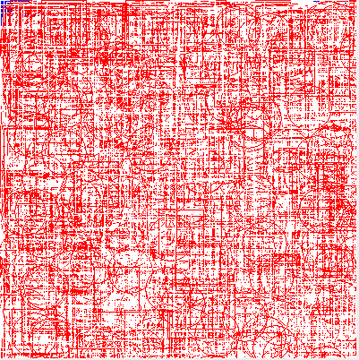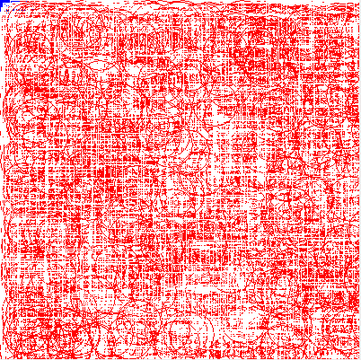posted on: 2011-06-03 16:17:04
I was exploring a question about threads and graphics and I wrote this simple test case.

>I created a test case to show two threads drawing to the same graphics object, afterwards I noticed a race condition. The simple solution is to create a lock via synchronized.

Here is a simple test case to show that two different threads can use the same graphics object.

```public class TestThreadedGraphics extends JPanel {
final BufferedImage drawing = new BufferedImage(400,400,BufferedImage.TYPE_INT_ARGB);

final Graphics g = drawing.getGraphics();

public void init(){
g.setColor(Color.BLUE);
g.fillRect(0,0,400,400);

//draw white squares.
final Random A = new Random();
public void run(){

for(;;){
int x = (int)(400*A.nextDouble());
int y = (int)(400*A.nextDouble());

g.setColor(Color.WHITE);
g.drawRect(x,y,50,50);

try{
} catch (InterruptedException e) {
//whatever
}
}

}
};
a.start();

//draw red circles.

final Random B = new Random();
public void run(){
for(;;){
int x = (int)(400*B.nextDouble());
int y = (int)(400*B.nextDouble());

g.setColor(Color.RED);
g.drawOval(x, y, 50, 50);

try{
} catch (InterruptedException e) {
//whatever
}
}

}
};
b.start();

}

@Override
public void paint(Graphics g){
g.drawImage(drawing,0,0,this);
}

public static void main(String[] args){
JFrame f = new JFrame("drawing threads");
f.setDefaultCloseOperation(JFrame.EXIT_ON_CLOSE);
f.setSize(500,500);
p.init();
p.setBounds(0,0,400,400);
f.setVisible(true);

for(;;){
f.repaint();
try{

} catch (InterruptedException e) {
e.printStackTrace();
}
}
}
}
```

So what this does is pretty simple, it creates a JFrame, with a panel and draws ad nauseum. What I want to point out is the race condition and the simple solution.Notice the red squares (and white circles), the red squares show that between setting the color, and painting the square the color changes. To alleviate this problem I have applied a simple solution. We need a lock, so that nothing else uses the graphics during the set color and draw operation.

To accomplish this I surrounded both graphics calls in a synchronized block.

```synchronized (g){
g.setColor(Color.RED);
g.drawOval(x, y, 50, 50);
}
```

By synchronizing on Graphics g we have essentially created a lock and removed a race condition. Further it should be noted that both threads are essentially modifying the BufferedImage drawing which creates another race condition with the paint method, that is being called from the EDT. This can be avoided by enclosing the drawImage call in a synchronized block.Now there are not any red squares, or white circles. One thing to note is that we do not need to use the Graphics object, we can use any object that the two threads have a reference to. In this case the graphics object is convenient since both threads have reference to it, but it can be any object.

Finally I have updated this post based on the comments of JC, who has also recommended the book Concurrent Programming in Javaâ„¢: Design Principles and Pattern (2nd Edition).